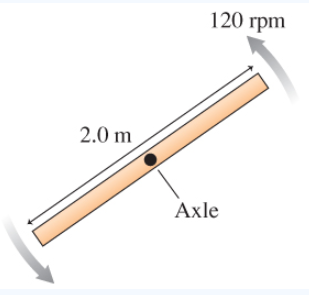# Problem: The 560 g bar is rotating as shown in (Figure 1).What is the angular momentum of the bar about the axle?Express your answer to two significant figures and include the appropriate units.L = ______

###### FREE Expert Solution

Moment of inertia of a rod about its center of mass:

$\overline{){\mathbit{i}}{\mathbf{=}}\frac{\mathbf{1}}{\mathbf{12}}{\mathbit{m}}{{\mathbit{l}}}^{{\mathbf{2}}}}$

Where m is mass and l is length

Angular momentum:

$\overline{){\mathbf{L}}{\mathbf{=}}{\mathbit{i}}{\mathbf{\omega }}}$

m = 560 g(1kg/1000g) = 0.56 kg

Length, l = 2.0m

98% (98 ratings)###### Problem Details

The 560 g bar is rotating as shown in (Figure 1).What is the angular momentum of the bar about the axle?

Express your answer to two significant figures and include the appropriate units.

L = ______